Next: Curl Up: Vectors Previous: Divergence

## The Laplacian

So far we have encountered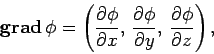(131)

which is a vector field formed from a scalar field, and(132)

which is a scalar field formed from a vector field. There are two ways in which we can combineand div. We can either form the vector field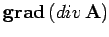or the scalar field. The former is not particularly interesting, but the scalar fieldturns up in a great many physics problems, and is, therefore, worthy of discussion.

Let us introduce the heat flow vector, which is the rate of flow of heat energy per unit area across a surface perpendicular to the direction of. In many substances, heat flows directly down the temperature gradient, so that we can write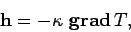(133)

whereis the thermal conductivity. The net rate of heat flow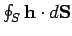out of some closed surfacemust be equal to the rate of decrease of heat energy in the volumeenclosed by. Thus, we can write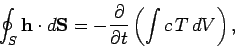(134)

where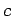is the specific heat. It follows from the divergence theorem that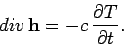(135)

Taking the divergence of both sides of Eq. (133), and making use of Eq. (135), we obtain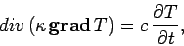(136)

or(137)

Ifis constant then the above equation can be written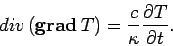(138)

The scalar fieldtakes the form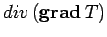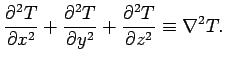(139)

Here, the scalar differential operator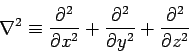(140)

is called the Laplacian. The Laplacian is a good scalar operator (i.e., it is coordinate independent) because it is formed from a combination of div (another good scalar operator) and(a good vector operator).

What is the physical significance of the Laplacian? In one dimension,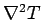reduces to. Now,is positive if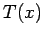is concave (from above) and negative if it is convex. So, if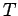is less than the average ofin its surroundings thenis positive, and vice versa.

In two dimensions,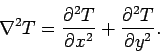(141)

Consider a local minimum of the temperature. At the minimum, the slope ofincreases in all directions, sois positive. Likewise,is negative at a local maximum. Consider, now, a steep-sided valley in. Suppose that the bottom of the valley runs parallel to the-axis. At the bottom of the valleyis large and positive, whereasis small and may even be negative. Thus,is positive, and this is associated withbeing less than the average local value.(142)
It is clear that ifis positive thenis locally less than the average value, so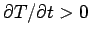: i.e., the region heats up. Likewise, ifis negative thenis locally greater than the average value, and heat flows out of the region: i.e.,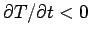. Thus, the above heat conduction equation makes physical sense.